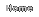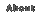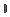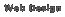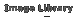function MM_preloadImages() { //v3.0 var d=document; if(d.images){ if(!d.MM_p) d.MM_p=new Array(); var i,j=d.MM_p.length,a=MM_preloadImages.arguments; for(i=0; i0&&parent.frames.length) { d=parent.frames[n.substring(p+1)].document; n=n.substring(0,p);} if(!(x=d[n])&&d.all) x=d.all[n]; for (i=0;!x&&i 2) { if ((img = MM_findObj(args)) != null && !img.MM_init) { img.MM_init = true; img.MM_up = args; img.MM_dn = img.src; if ((nbArr = document[grpName]) == null) nbArr = document[grpName] = new Array(); nbArr[nbArr.length] = img; for (i=4; i